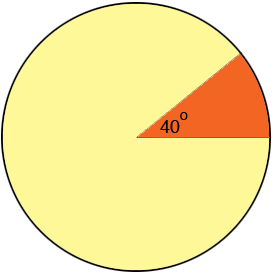SEARCH HOMEMath Central Quandaries & QueriesQuestion from Bill, a student: Hi,the Central Angle of a sector of a circle is 40 degrees. The circular arc of the curve has a chord length of 3000 ft. Find the radius(r) of the circular arc.Hi Bill,

The circumference of a circle is 2 π r where r is the radius. The length of the circular arc is only a fraction of the circumference because the central angle of 40 degrees is only a fraction of a complete revolution, 360 degrees.Since 360/40 = 9 it takes 9 of these sectors to fill the circle and thus the circumference must be 9 times the chord length. Hence

2 π r = 9 × 3000 feet.

What is r?

PennyMath Central is supported by the University of Regina and The Pacific Institute for the Mathematical Sciences.